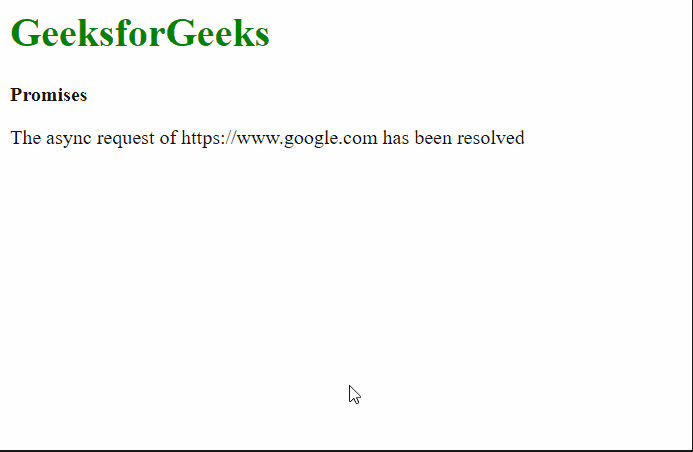# How to run a given array of promises in series in JavaScript ?

Given an array of Promises, we have to run that in a series. To do this task, we can use then(), to run the next promise, after the completion of a promise.

Approach: The then() method returns a Promise, which helps us to chain promises/methods. The Promise.resolve() method executes the first callback, and when this promise is fulfilled, then it passes to the next function callback1, and it goes on until all the promises are fulfilled. In this way, we can run all the Promises in series.

Syntax:

```Promise.resolve( callback0 )
.then( callback1 )
.then( callback2 )
.then( callback3 )...    ```

Example 1: In this example, we will be executing 3 promises by chaining each of them with the then() method.

## HTML

 `<``html``> ` `<``body``> ` `  ``<``h1` `style``=``"color: green;"``> ` `    ``GeeksforGeeks ` `  `` ` `  ``<``b``>Promises ` ` `  `  ``<``script``> ` `    ``// Define an asynchronous function, ` `    ``// results in returning a promise ` `    ``async function task(url) { ` `      ``console.log(url + " will be fetched now") ` ` `  `      ``return fetch(url); ` `    ``} ` ` `  `    ``// Declaring an array of URLs ` `    ``let arr = [ ` `      ``"https://www.google.com", ` `      ``"https://www.facebook.com", ` `      ``"https://www.twitter.com" ` `    ``]; ` ` `  `    ``// Resolving the first task ` `    ``Promise.resolve(() => { ` `      ``return task(arr); ` `    ``}) ` ` `  `    ``// Resolving the second task ` `      ``.then(() => { ` `        ``return task(arr); ` `      ``}) ` ` `  `    ``// Resolving the third task ` `      ``.then(() => { ` `        ``return task(arr); ` `      ``}); ` `  `` ` ` ` ``

Output:

```https://www.facebook.com will be fetched now

The above approach is not feasible if there are a lot more promises in the array. Chaining of the function would get very tiring and will make the code lengthy. We can use the forEach() array function to execute the promises by storing the results in a variable and updating that variable at every promise. This will automatically go through all the promises and one can prevent repeatedly writing then() statements.

Example 2: In this example, we will be executing multiple promises by using the forEach() method.

## HTML

 `<``html``> ` `<``body``> ` `  ``<``h1` `style``=``"color: green;"``> ` `      ``GeeksforGeeks ` `  `` ` `  ``<``b``>Promises ` `  ``<``script``> ` ` `  `    ``// Define an asynchronous function ` `    ``async function task(url) { ` `      ``return fetch(url); ` `    ``} ` ` `  `    ``// Define array that has to be processed ` `    ``// by the asynchronous function ` `    ``let arr = [ ` `      ``"https://www.google.com", ` `      ``"https://www.facebook.com", ` `      ``"https://www.twitter.com", ` `      ``"https://www.youtube.com", ` `      ``"https://www.netflix.com", ` `    ``]; ` ` `  `    ``// Declare a Promise using its resolve constructor ` `    ``let promise = Promise.resolve(); ` ` `  `    ``// Use the forEach function to evaluate ` `    ``// the promises in series ` `     `  `    ``// The value of ` `    ``// p would be the result ` `    ``// of previous promise ` `    ``arr.forEach((url, index) => { ` `      ``promise = promise.then(() => { ` ` `  `        ``let para = document.createElement("p"); ` `        ``para.innerText =  ` `          ``"The async request of " + url + ` `          ``" has been resolved"; ` ` `  `        ``document.body.appendChild(para); ` ` `  `        ``return task(url); ` `      ``}); ` `    ``}); ` `  `` ` ` ` ``

Output:Whether you're preparing for your first job interview or aiming to upskill in this ever-evolving tech landscape, GeeksforGeeks Courses are your key to success. We provide top-quality content at affordable prices, all geared towards accelerating your growth in a time-bound manner. Join the millions we've already empowered, and we're here to do the same for you. Don't miss out - check it out now!

Previous
Next